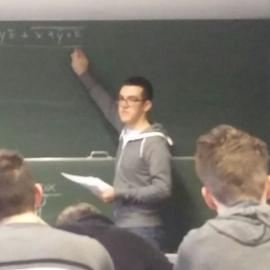# Short Exercise: Transfinite Closure in Analysis

Recently in the ##math chat on Freenode, somebody asked for some intuition and help on the following analysis problem:

Let $a < b$ be reals and $M \subseteq [a, b]$ a subset with these properties:

1. $a \in M$
2. For all non-decreasing sequences $(x_n)_n$ with a limit $x := \lim x_n$ (in $\mathbb{R}$), we have $x \in M$
3. For every $y \in M$ there is a $\delta > 0$ such that $(y - \delta, y + \delta) \cap [a, b] \subseteq [a, b]$

Prove that $M = [a, b]$.

It was quite a nice exercise for keeping my skills sharp: the claim is intuitively clear and can be proven more beautifully and less technically than one might think on first sight!

Update: I stumbled upon the post A principle of mathematical induction for partially ordered sets with infima? on MathOverflow, which presents a generalized induction principle very close to the instantiation I use below.

## The Intuition

• Property (2) is quite similar to stating that $M$ is closed in the subspace topology on $[a, b]$ except that it’s only upwards-closed.

• Property (3) simply says that $M$ is open in $[a, b]$.

• Let’s try a few sample $M$s:

• $M = [0, ½)$: contradicts (2)
• fixing that, $M = [0, ½]$: contradicts (3)
• fixing that, $M = [0, ½ + \delta)$: contradicts (2)
• fixing that, $M = [0, ½ + \delta]$: contradicts (3)
• $\ldots$

These iterations seem to hit the nail, but only informally so far. There is a bit of uncertainty whether $½ + \delta + \delta’ + \ldots$ really approaches $b$ after some transfinite amount of steps. In other words, we could see $[a, b]$ as the transfinite closure of $\varnothing$ wrt. properties (1)-(3).

Morally, these iterations always take the longest strip so far and make that even longer: if the longest strip so far is $[a, c]$, then by (3) make it into $[a, c + \delta)$ and in turn by (2) into $[a, c + \delta]$. That reminded me heavily of a partial order and of Zorn’s lemma! Indeed, it turns out that this leads to a correct formal proof as elaborated below.

## The Formalism

To make the proof smoother and less notation-heavy, let us rephrase property (2) a bit:

Definition: We call a subspace $M \subseteq \mathbb{R}$ upwards-closed if all non-decreasing Cauchy sequences in $M$ have a limit in $M$.

Now we can state the poset of strips and prove the existence of a maximal element by Zorn’s lemma:

Definition (Poset of Strips): For a pointed subset $(M, m) \subseteq \mathbb{R}$ define a partial order on the set of strips $[m, \cdot]$ with $\cdot \in M$ such that $$[m, m_1] \sqsubseteq [m, m_2] :\Leftrightarrow m_1 \leq m_2.$$

Lemma: Upwards-closed pointed subsets $(M, m)$ have a longest strip $[m, m’] \in M$.

Proof: Apply Zorn’s lemma. Let $T = (m_i)_{i \in I}$ be a chain in the poset of strips. If $T$ is empty, then $[m, m]$ is a trivial upper bound. Otherwise, define $m’ := \sup m_i$. If in fact $m’ \in T$ is an attained maximum, $[m, m’]$ is an upper bound of the chain. Otherwise, $[m, m’) \in M$ and by upwards-closedness $[m, m’] \in M$ is an upper bound.

And finally, we can elegantly1 prove the overall claim:

Theorem: Let $a < b$ be two real numbers and $(M, a)$ a subset $M \subseteq [a, b]$ that is open and upwards-closed therein. Then $M = [a, b]$.

Proof: The previous lemma guarantees a longest strip $[a, c] \subseteq M$. We show $c = b$ by assuming the contrary and deriving a contradiction. Since $M$ is open, there is $\delta > 0$ such that $[a, c + \delta) \subseteq M$. Due to upwards-closedness, we conclude that $[a, c + \delta] \subseteq M$ is an even longer strip contradicting the maximality given by the lemma.

1. Well, for some people it might be debatable how elegant (constructive) an invocation of Zorn’s lemma is. ^##### Navid Roux
###### Computer Science M. Sc. Student

Academically interested in formal systems for knowledge representation; recreationally in love with sports.